Example Questions

Example Question #81 : Coordinate Geometry

Which of the following equations represents a line that goes through the pointand is perpendicular to the line?Explanation:

In order to solve this problem, we need first to transform the equation from standard form to slope-intercept form:Transform the original equation to find its slope.First, subtractfrom both sides of the equation.Simplify and rearrange.Next, divide both sides of the equation by 6.The slope of our first line is equal to. Perpendicular lines have slopes that are opposite reciprocals of each other; therefore, if the slope of one is x, then the slope of the other is equal to the following:Let's calculate the opposite reciprocal of our slope:The slope of our line is equal to 2. We now have the following partial equation:We are missing the y-intercept,. Substitute the x- and y-values in the given pointto solve for the missing y-intercept.Add 4 to both sides of the equation.Substitute this value into our partial equation to construct the equation of our line:Example Question #3 : How To Find The Equation Of A Perpendicular Line

What is the equation of a line that runs perpendicular to the line 2x + = 5 and passes through the point (2,7)?

x/2 + y = 6

2x + y = 7

x/2 – y = 6

2x – y = 6

x/2 + y = 5

x/2 + y = 6

Explanation:

First, put the equation of the line given into slope-intercept form by solving for y. You get y = -2x +5, so the slope is –2. Perpendicular lines have opposite-reciprocal slopes, so the slope of the line we want to find is 1/2. Plugging in the point given into the equation y = 1/2x + b and solving for b, we get b = 6. Thus, the equation of the line is y = ½x + 6. Rearranged, it is –x/2 + y = 6.

Example Question #1 : How To Find The Slope Of Perpendicular Lines

Two points on line m are (3,7) and (-2, 5).  Line k is perpendicular to line m.  What is the slope of line k?

2/5

-5

0

-5/2

3

-5/2

Explanation:

The slope of line m is the (y2 - y1) / (x- x1) = (5-7) / (-2 - 3)

= -2 / -5

= 2/5

To find the slope of a line perpendicular to a given line, we must take the negative reciprocal of the slope of the given line.

Thus the slope of line k is the negative reciprocal of 2/5 (slope of line m), which is -5/2.

Example Question #2 : How To Find The Slope Of Perpendicular Lines

The equation of a line is: 8x + 16y = 48

What is the slope of a line that runs perpendicular to that line?

2

-1/4

-2

-1/8

8

2

Explanation:

First, solve for the equation of the line in the form of y = mx + b so that you can determine the slope, m of the line:

8x + 16y = 48

16y = -8x + 48

y = -(8/16)x + 48/16

y = -(1/2)x + 3

Therefore the slope (or m) = -1/2

The slope of a perpendicular line is the negative inverse of the slope.

m = - (-2/1) = 2

Example Question #3 : How To Find The Slope Of Perpendicular Lines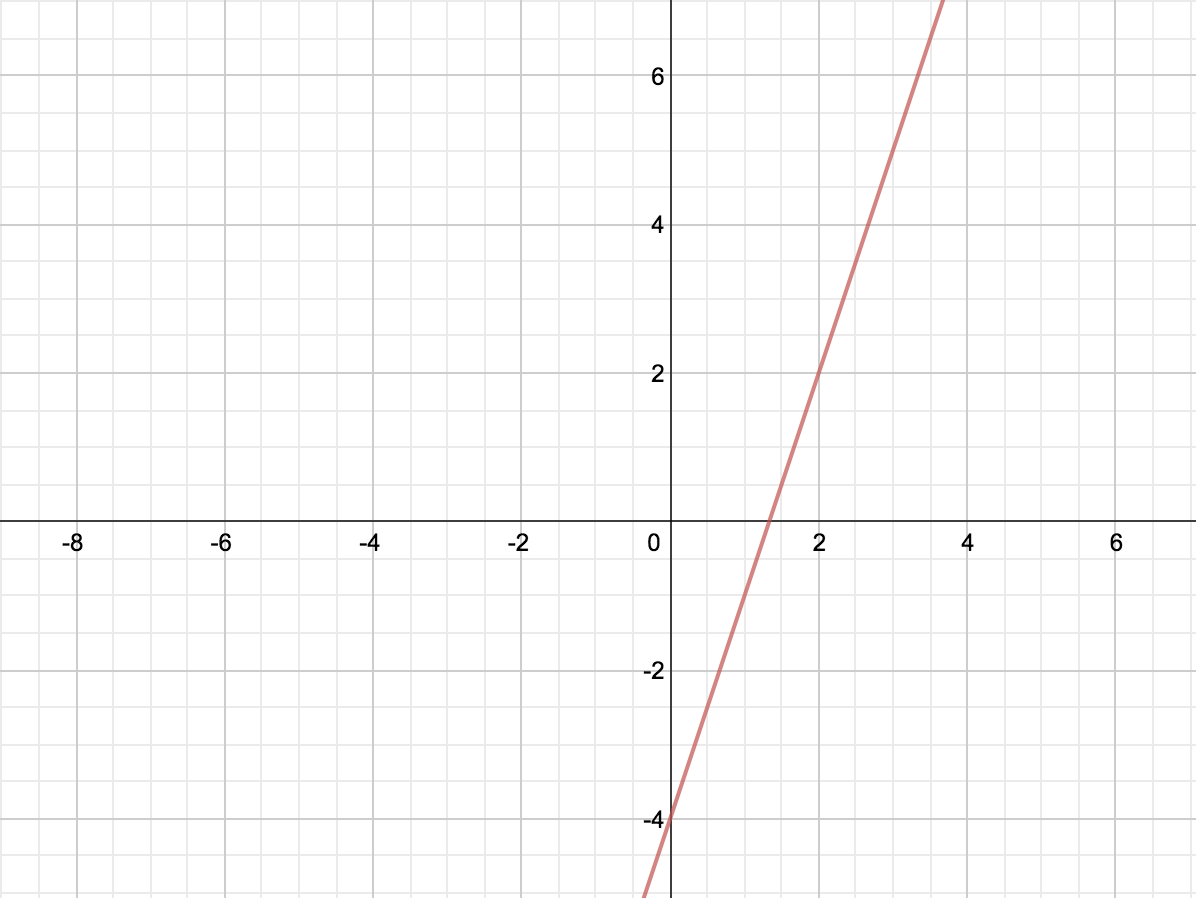What is the equation of a line perpendicular to the one above, passing through the point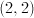?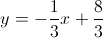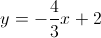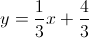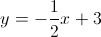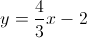Explanation:

Looking at the graph, we can tell the slope of the line is 3 with a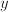-intercept of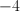, so the equation of the line is: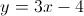A perpendicular line to this would have a slope of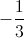, and would pass through the pointso it follows: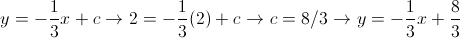Example Question #4 : How To Find The Slope Of Perpendicular Lines

Line M passes through the points (2,2) and (3,–5).  Which of the following is perpendicular to line M?

y = –(1/7)x – 1

y = 7x + 4

y = 7x – 6

y = –7x – 5

y = (1/7)x + 3

y = (1/7)x + 3

Explanation:

First we find the slope of line M by using the slope formula (y– y1)/(x– x1).

(–5 – 2)/(3 – 2) = –7/1. This means the slope of Line M is –7.  A line perpendicular to Line M will have a negative reciprocal slope. Thus, the answer is = (1/7)x + 3.

Example Question #61 : Coordinate Geometry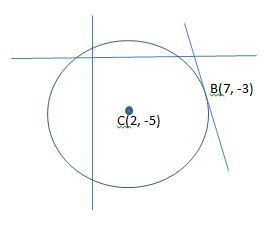Figure not drawn to scale.

In the figure above, a circle is centered at point C and a line is tangent to the circle at point B. What is the equation of the line?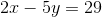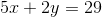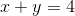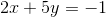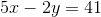Explanation:

We know that the line passes through point B, but we must calculate its slope in order to find the equation that defines the line. Because the line is tangent to the circle, it must make a right angle with the radius of the circle at point B. Therefore, the slope of the line is perpendicular to the slope of the radius that connects the center of the circle to point B. First, we can find the slope of the radius, and then we can determine the perpendicular slope.

The radius passes through points C and B. We can use the formula for the slope (represented as) between two points to find the slope of the radius.

Point C: (2,-5) and point B: (7,-3)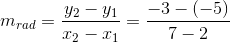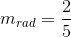This is the slope of the radius, but we need to find the slope of the line that is perpendicular to the radius. This value will be equal to the negative reciprocal.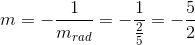Now we know the slope of the tangent line. We can use the point-slope formula to find the equation of the line. The formula is shown below.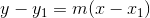Plug in the give point that lies on the tangent line (point B) and simplify the equation.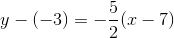Multiply both sides by two in order to remove the fraction.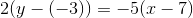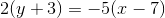Distribute both sides.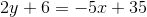Add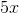to both sides.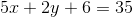Subtract six from both sides.The answer is.

Example Question #1 : How To Find The Slope Of A Perpendicular Line

What is the slope of any line perpendicular to 2y = 4x +3 ?

– ½

– 4

2

½

– ½

Explanation:

First, we must solve the equation for y to determine the slope:  y = 2x + 3/2

By looking at the coefficient in front of x, we know that the slope of this line has a value of 2. To fine the slope of any line perpendicular to this one, we take the negative reciprocal of it:

slope = m , perpendicular slope = – 1/m

slope = 2 , perpendicular slope = – 1/2

Example Question #2 : How To Find The Slope Of A Perpendicular Line

What line is perpendicular to 2x + y = 3 at (1,1)?

2x + 3y = 1

x – 2y = -1

3x + 2y = 1

x + 2y - 3

x – 2y = -1

Explanation:

Find the slope of the given line.  The perpendicular slope will be the opposite reciprocal of the original slope.  Use the slope-intercept form (y = mx + b) and substitute in the given point and the new slope to find the intercept, b.  Convert back to standard form of an equation:  ax + by =  c.

Example Question #3 : How To Find The Slope Of A Perpendicular Line

What is the slope of the line perpendicular to the line given by the equation

6x – 9y +14 = 0

-1/6

2/3

-3/2

6

-2/3